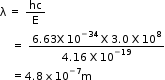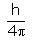NEET  >  Test: Dual Nature of Matter

# Test: Dual Nature of Matter

Test Description

## 10 Questions MCQ Test Chemistry Class 11 | Test: Dual Nature of Matter

Test: Dual Nature of Matter for NEET 2023 is part of Chemistry Class 11 preparation. The Test: Dual Nature of Matter questions and answers have been prepared according to the NEET exam syllabus.The Test: Dual Nature of Matter MCQs are made for NEET 2023 Exam. Find important definitions, questions, notes, meanings, examples, exercises, MCQs and online tests for Test: Dual Nature of Matter below.
Solutions of Test: Dual Nature of Matter questions in English are available as part of our Chemistry Class 11 for NEET & Test: Dual Nature of Matter solutions in Hindi for Chemistry Class 11 course. Download more important topics, notes, lectures and mock test series for NEET Exam by signing up for free. Attempt Test: Dual Nature of Matter | 10 questions in 10 minutes | Mock test for NEET preparation | Free important questions MCQ to study Chemistry Class 11 for NEET Exam | Download free PDF with solutions
 1 Crore+ students have signed up on EduRev. Have you?
Test: Dual Nature of Matter - Question 1

### A photon has energy of 2.6 eV. What is its wavelength?

Detailed Solution for Test: Dual Nature of Matter - Question 1

Given, 2.6 e V = 2.6 X 1.6 X 10-19 J = 4.16 X 10-19 J

Now,by using formula,Test: Dual Nature of Matter - Question 2

### If uncertainty in the position of an electron is zero, the uncertainity in its momentum would be

Detailed Solution for Test: Dual Nature of Matter - Question 2

Actually, according to uncertainty principle,

Δx.Δp ≥ h/4π

or Δx.Δp ≈ h/4n

where, Δx, is uncertainty in position and Δp is uncertainty in momentum.

So, if Δx = 0

Hence, uncertainty in momentum Δp = h/4π x 0 = Infinity.

Test: Dual Nature of Matter - Question 3

### Uncertainty in the position of an electron (mass 9.1 x 10-31 kg) moving with a velocity of 300 ms-1, accurate upto 0.001% will be:

Detailed Solution for Test: Dual Nature of Matter - Question 3

Change in position (X),
P= momentum,
P=mv.
X ×P = h/4π.
X× m v = h/4π.
X= h/4πmv.
X=6.625×10-34/4 ×3.14×9.1×10^-31×300.
X=6.625×10-5/342.8.
X=0.0192×10^-5.
X= 19.2× 10-7.
in question it is given to take accurate up to 0.001%.= 1×10-3/100=1 ×10-5.
X= 19.2 ×10-7/10-5.
X=19.2 × 10-2.

Test: Dual Nature of Matter - Question 4

What experimental result did Albert Einstein cite as evidence that light has particle-like properties?

Detailed Solution for Test: Dual Nature of Matter - Question 4

Einstein's explanation of photoelectric effect using the concept of photons. In 1905, Albert Einstein provided an explanation of the photoelectric effect, an experiment that the wave theory of light failed to explain. He did so by postulating the existence of photons, quanta of light energy with particulate qualities.

Test: Dual Nature of Matter - Question 5

The de Broglie wavelength of a particle is given by.

Detailed Solution for Test: Dual Nature of Matter - Question 5

According to De Broglie, a moving small particle like electron, proton, neutron, dust particle, a small ball etc. has the properties of a wave (Dual nature of matter).

The wave length of a moving particle may be calculated as

λ=h/mv =h/p

De Broglie equation

λ =wave length

h= planck’s constt.

m=mass of particle

v=velocity of particle

p=momentum of the particle

Test: Dual Nature of Matter - Question 6

The de – Broglie wavelength of an electron is 600 nm. The velocity of the electron having the mass 9.1 X 10-31 Kg is

Detailed Solution for Test: Dual Nature of Matter - Question 6

1nm= 10^-9m.
wavelength= 600nm= 600 ×10-9m
wavelength= h/p.
wavelength= h/mv
v= h/ m wavelength
v=6.625×10^-34/9.1×10^-31×600× 10-9.
v= 6.625 × 10+4/9.1 ×6.
v= 6.625× 10+4/9.1×6.
v= 6.625 ×104/54.6.
v= 0.12 × 104.
v= 0.0012 × 10+6 .

Test: Dual Nature of Matter - Question 7

In the relationship ∆x. ∆p =, ∆p is:

Detailed Solution for Test: Dual Nature of Matter - Question 7

The uncertainty principle is alternatively expressed in terms of a particle’s momentum and position. The momentum of a particle is equal to the product of its mass times its velocity. Thus, the product of the uncertainties in the momentum and the position of a particle equals h/(4π)

Test: Dual Nature of Matter - Question 8

The uncertainty principle states that

Detailed Solution for Test: Dual Nature of Matter - Question 8

The Heisenberg uncertainty principle states that it is impossible to know simultaneously the exact position and momentum of a particle. That is, the more exactly the position is determined, the less known the momentum, and vice versa.

Test: Dual Nature of Matter - Question 9

Whch of the following will have the maximum value of ∆v . ∆x

Detailed Solution for Test: Dual Nature of Matter - Question 9

This is accordance with the Heisenberg's uncertainty principle.It states that it is not possible to accurately determine the position and momentum of a microscopic particle such as electron simultaneously.

Test: Dual Nature of Matter - Question 10

The de Broglie wavelength of an electron is 8.7 x 10-11 m. The mass of an electron is 9.1 x 10-31 kg. The velocity of this electron is:

Detailed Solution for Test: Dual Nature of Matter - Question 10

Wavelength = h/p = h/ mv.
v= h/ m.wavelength
v= 6.625 × 10-34/9.1×10-31 × 8.7 × 10-11
v= 6.625 × 108/ 9.1× 8.7
v= 0.08367 ×108.

## Chemistry Class 11

204 videos|331 docs|229 tests
 Use Code STAYHOME200 and get INR 200 additional OFF Use Coupon Code
Information about Test: Dual Nature of Matter Page
In this test you can find the Exam questions for Test: Dual Nature of Matter solved & explained in the simplest way possible. Besides giving Questions and answers for Test: Dual Nature of Matter, EduRev gives you an ample number of Online tests for practice

## Chemistry Class 11

204 videos|331 docs|229 tests

### How to Prepare for NEET

Read our guide to prepare for NEET which is created by Toppers & the best Teachers